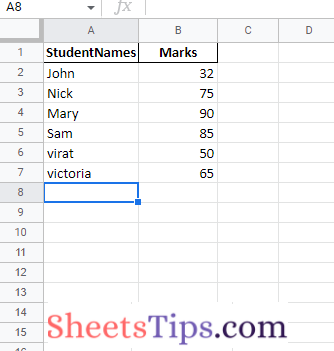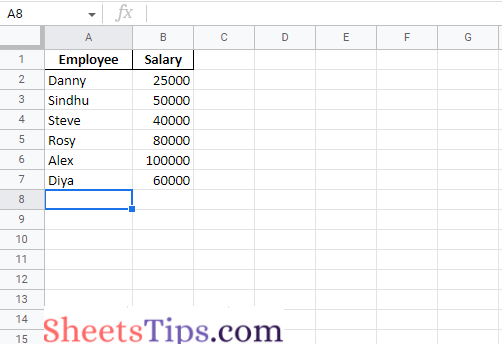# How to Write Pandas Dataframes for Multiple Sheets in Excel Using ExcelWriter()?

To export different data frames to different Excels using Python, we must use the xlsxwriter function offered by Pandas. ExcelWriter() is a class that allows DataFrame objects to be written into Microsoft Excel sheets. ExcelWriter() supports the creation of text, integers, strings, and formulas and It may also be used for several spreadsheets. Let us explore everything about ExcelWriter() and how to write pandas data frames for multiple sheets in an excel file. Read further to find out more.

## ExcelWriter() Function

Pandas have a function called xlsxwriter for this. ExcelWriter() is a class that allows DataFrame objects to be written into Microsoft Excel sheets. ExcelWriter() supports the creation of text, integers, strings, and formulas. It can also be used for several spreadsheets.

Syntax:

`pandas.ExcelWriter(path, date_format=None, mode='w')`

Parameters

path: It is the path of the xls or xlsx or ods file given as input in a string format.

date_format: It is the Date format string for Excel files (for example, ‘YYYY-MM-DD’). The default is  None.

mode: It may be {‘w’, ‘a’}. The default is ‘w’. It is the file mode that we want to use(write/append). Append does not support fsspec URLs.

Python to_excel() Function:

To export the DataFrame to an excel file, use the to_excel() method. The target file name must be specified when writing a single object to an excel file. If we wish to write to multiple sheets, we must first create an ExcelWriter object with the target filename and then indicate the sheet in the file we want to write to. The unique sheet_name can also be used to write multiple sheets. All modifications made to the data written to the file must be saved.

Syntax:

`DataFrame.to_excel(excel_writer, sheet_name='Sheet1', index=True)`

Parameters

excel_writer: It is the file path/file, or ExcelWriter object (new or existing)

sheet_name: It is the name of the sheet containing DataFrame. ‘Sheet1’ by default. It is in string format.

index: It is used to write row names (index). This is boolean and True by default.

## Python Code to Write Pandas DataFrames to Multiple Excel Sheets

Approach:

• Import the pandas module using the import keyword
• Create a first dataframe passing some random a dictionary(where values are stored as a list) as an argument to the DataFrame() function of the pandas module.
Store it in a variable.
• Create a second dataframe passing some random a dictionary(where values are stored as a list)
as an argument to the DataFrame() function of the pandas module.
Store it in another variable.
• Similarly, create the third dataframe and store it in another variable.
• Print the given first dataframe.
Print the given second dataframe.
Print the given third dataframe.
• Create an excel writer object by passing the file path as an argument to the ExcelWriter() function
• Pass the above excel writer object, sheet name, index=False to the use to_excel() function
to store the above-given dataframes in specified sheets.
• The Exit of the Program.

Below is the implementation:

```# Import the pandas module using the import keyword
import pandas as pd

# Create a first dataframe passing some random a dictionary(where values are stored as a list)
# as an argument to the DataFrame() function of the pandas module.
# Store it in a variable.
gvn_datafrme1 = pd.DataFrame({'StudentNames': ['John', 'Nick', 'Mary',
'Sam', 'virat', 'victoria'],
'Marks': [32, 75, 90, 85, 50, 65]})

# Create a second dataframe passing some random a dictionary(where values are stored as a list)
# as an argument to the DataFrame() function of the pandas module.
# Store it in another variable.
gvn_datafrme2 = pd.DataFrame({'Websites': ['SheetsTips', 'Python-Programs', 'BtechGeeks',
'About': ['Excel', 'Python codes', 'All courses', 'Arrays', 'Browser', 'Social-media App']})

# Similarly create the third dataframe and store it in another variable.
gvn_datafrme3 = pd.DataFrame({'Countries': ['India', 'USA', 'France',
'Australia', 'Srilanka', 'China'],
'Capitals': ['New Delhi', 'Washington.DC', 'Paris', 'Canberra', 'Colombo', 'Beijing']})

# Print the given first dataframe
print(gvn_datafrme1)
print()
# Print the given second dataframe
print(gvn_datafrme2)
print()
# Print the given third dataframe
print(gvn_datafrme3)

# Create an excel writer object by passing the filepath as an argument to the ExcelWriter() function
with pd.ExcelWriter("Output_Excelfile.xlsx") as xlwriter:

# Pass the above excel writer object, sheet name, index=False to the use to_excel() function
# to store the above given dataframes in a specified sheets.
gvn_datafrme1.to_excel(xlwriter, sheet_name="StudentNames&Marks", index=False)
gvn_datafrme2.to_excel(xlwriter, sheet_name="Websites", index=False)
gvn_datafrme3.to_excel(xlwriter, sheet_name="Countries", index=False)
```

Output:

```  StudentNames  Marks
0         John     32
1         Nick     75
2         Mary     90
3          Sam     85
4        virat     50
5     victoria     65

0       SheetsTips             Excel
1  Python-Programs      Python codes
2       BtechGeeks       All courses
3      PythonArray            Arrays
4           Chrome           Browser

Countries       Capitals
0      India      New Delhi
1        USA  Washington.DC
2     France          Paris
3  Australia       Canberra
4   Srilanka        Colombo
5      China        Beijing```

Output Image:Explanation:

`Here, multiple sheets were saved in the specified location in an Excel file.`

### Another way of storing the Dataframe in an Existing Excel file using ExcelWriter() function

Here we append a new excel sheet to the already existing Excel file instead of creating a new file.

We now use the above Output_Excelfile and append a new sheet to that file by passing the argument mode as ‘a’ (append).

Using mode ‘a,’ the new sheet will be added as the last sheet in the existing excel file.

Approach:

• Import the pandas module using the import keyword
• Create a dataframe passing some random a dictionary(where values are stored as a list) as an argument to the DataFrame() function of the pandas module.
• Store it in a variable.
Print the given dataframe.
• Create an excel writer object by passing the filepath, mode as append, and engine=”openpyxl” as an argument to the ExcelWriter() function.
• Here it is used to append the given 4th dataframe as a last sheet in the existing Excel file
Pass the above excel writer object, sheet name, index=False to the use to_excel() function
to store the above-given dataframe in a specified sheet.
• Here we are appending a new sheet(Employee&salary) to the already existing Output_Excelfile

Below is the implementation:

```# Import the pandas module using the import keyword
import pandas as pd

# Create a dataframe passing some random a dictionary(where values are stored as a list)
# as an argument to the DataFrame() function of the pandas module.
# Store it in a variable.
gvn_datafrme4 = pd.DataFrame({'Employee': ['Danny', 'Sindhu', 'Steve',
'Rosy', 'Alex', 'Diya'],
'Salary': [25000, 50000, 40000, 80000, 100000, 60000]})

# Print the given dataframe
print(gvn_datafrme4)

# Create an excel writer object by passing the filepath, mode as append and engine="openpyxl" as an argument
# to the ExcelWriter() function.
# Here it is used to append the given 4th dataframe as a last sheet in the existing Excel file
with pd.ExcelWriter("Output_Excelfile.xlsx", mode="a", engine="openpyxl") as writer:

# Pass the above excel writer object, sheet name, index=False to the use to_excel() function
# to store the above given dataframe in a specified sheet.
# Here we are appending a new sheet(Employee&salary) to the already existing Output_Excelfile
gvn_datafrme4.to_excel(writer, sheet_name="Employee&salary", index=False)
```

Output:

```  Employee  Salary
0    Danny   25000
1   Sindhu   50000
2    Steve   40000
3     Rosy   80000
4     Alex  100000
5     Diya   60000```The Pandas ExcelWriter () function is one of the most useful functions for writing data frames for multiple sheets. But to get this done in Excel, make sure you are installing xlsxwriter.